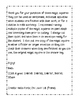# Magic Squares: Addition, Subtraction, Multiplication, and DivisionSubject
Resource Type
File Type
PDF (440 KB|8 pages)
Standards
\$3.00
• Product Description
• Standards
These Magic Squares are an excellent addition to any math workshop station or as an extension activity for the student who finishes their work early. The Magic Squares contain problems for addition, subtraction, missing multiplier/ multiplicand, and dividends activities for 2nd and 3rd grade classes. I would recommend running these off on card stock and laminating prior to use. Placing them in an envelope for the student to use when needed and placed at a station in the classroom makes for an easy activity for students and an easy to use activity for you to arrange. Placing the answer key in a folder near by for the students to check their own progress helps them to take ownership in their own learning.
Determine the unknown whole number in a multiplication or division equation relating three whole numbers. For example, determine the unknown number that makes the equation true in each of the equations 8 × ? = 48, 5 = __ ÷ 3, 6 × 6 = ?.
Fluently add and subtract within 1000 using strategies and algorithms based on place value, properties of operations, and/or the relationship between addition and subtraction.
Add and subtract within 1000, using concrete models or drawings and strategies based on place value, properties of operations, and/or the relationship between addition and subtraction; relate the strategy to a written method. Understand that in adding or subtracting three-digit numbers, one adds or subtracts hundreds and hundreds, tens and tens, ones and ones; and sometimes it is necessary to compose or decompose tens or hundreds.
Fluently add and subtract within 100 using strategies based on place value, properties of operations, and/or the relationship between addition and subtraction.
Read and write numbers to 1000 using base-ten numerals, number names, and expanded form.
Total Pages
8 pages